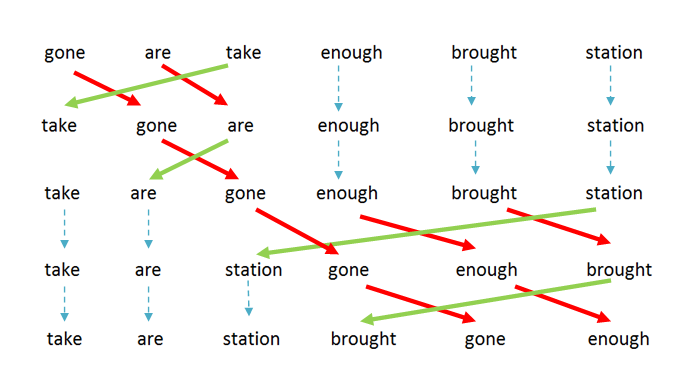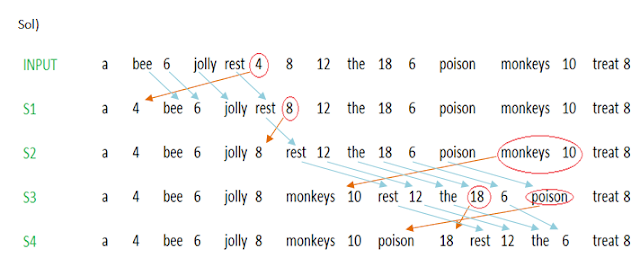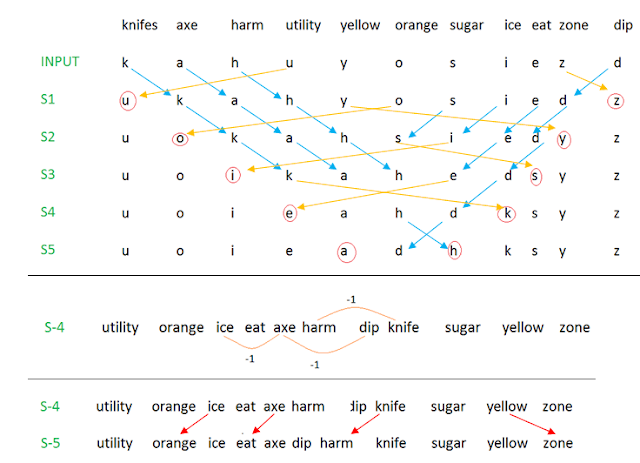# Reasoning Ability: Input Output Tricks with ExamplesInput and Output is an important section in Bank PO/SO exams. It covers 5 to 7 marks in the reasoning section. But most of the candidates, try to avoid this section as it is time consuming and tricky. So, today we have provided you the different types of sequences present in this section.

## What is Input and Output?

In this type of questions, we are given with an Input. The Input might be number series, alphabet series or an alpha-numeric series arranged in a jumbled form. The Input is then followed by various steps resulting in an outcome. It is similar to a flow-chart or an algorithm explaining how an output is obtained from any given input

The first step in solving these types of questions is to determine the pattern

### Models : Patterns to look for in the given sequence are

• Arranging the given words in forward/reverse alphabetical order
• Arranging the given numbers in ascending/descending order
• Changing places of words/numbers according to a set pattern
• Arithmetic Operations-Different steps are obtained by taking the numbers of the input and different arithmetic operations are performed after that
• Miscellaneous

## Example No 1

### Alphabetical Series (Model 1 )

A word arrangement machine, when given an input line of words, rearranges it in every step following a certain rule. Following is an illustration of an input line of words and various steps of rearrangement.
INPUT : gone are take enough brought station
STEP 1 : take gone are enough brought station
STEP 2 : take are gone enough brought station
STEP 3 : take are station gone enough brought
STEP 4 : take are station brought gone enough

• And, step-4 is the last step for this input. Now, find out an appropriate step in each of the following questions following the above rule.

#### Explanation:

• As I have mentioned earlier, the 1st step in solving such problems, is to determine the pattern. For pattern determination, always observe the Input and the Last step.
• If we observe the last step carefully, we find that the words are written in alternative forward/reverse alphabetical order.
• The alphabetical order of these words as per the dictionary is - are, brought, enough, gone, station, take.

The alphabetical position of the 1st letter of each word
A =1
B=2
E=5
G=7
S=19
T=20• In the steps, the word which comes alphabetically last in position is shifted in the 1st position and the remaining words are shifted right.
• Then, the word which comes alphabetically first in position is shifted to the 2nd position and the remaining words are shifted right and so on, till the desired pattern is obtained.
• We can make use of this technique to determine the pattern (or)we can number each word and follow the number pattern to determine the answers.## Now let’s just focus on the numbers and try to solve the problem

Ques 1 INPUT: car on star quick demand fat.
What will be the third step for this input?
(a) Star quick car demand on fat
(b) Star car quick demand on fat
(c) Star car demand quick on fat
(d) Star car quick on demand fat
(e) None of these## Example 2 (Model No-2 )

A number sorting machine when given an input if numbers, rearranges them in a particular manner step-by-step as indicated below till all the numbers are arranged. Given below is an illustration of this arrangement.

INPUT : 39 121 48 18 76 112 14 45 63 96STEP-1 : 14 39 121 48 18 76 112 45 63 96
STEP-2 : 14 39 48 18 76 112 45 63 96 121
STEP-3 : 14 18 39 48 76 112 45 63 96 121
STEP-4 : 14 18 39 48 76 45 63 96 112 121
STEP-5 : 14 18 39 45 48 76 63 96 112 121
STEP-6 : 14 18 39 45 48 63 76 96 112 121

Answer:  STEP-6 is the last step for this input.

## Explanation

• The last step indicates that the numbers are arranged in an ascending order.
• In the 1st step , the smallest number is placed in the first position and the remaining numbers are shifted.
• In the 2nd step, the largest number is placed in the last position and the remaining numbers are shifted. So, alternatively the numbers are arranged in ascending position and if the number is already present in the desired position, then it is left un-shifted.### Ques 1

How many steps will be required for getting the final output for the following input?
INPUT : 101 85 66 49 73 39 142 25 115 74Ans) 8 steps are required

### Ques 2

Given below is the 5th step of an input. What will be the 3rd step?STEP-5: 17 32 43 82 69 93 49 56 99 106
(A)17 32 82 43 69 93 49 56 99 106
(B) 17 32 82 69 43 93 49 56 99 106
(C) 17 32 82 69 93 43 49 56 99 106
(D) 17 32 82 69 43 93 56 49 99 106
(E) Cannot be determined

Ans) Since the numbers can be arranged in any possible way, it is not possible to determine any of the previous steps.

### Ques 3

INPUT : shop 17 table 20 53 oven desk 39
STEP1 : 17 shop table 20 53 oven desk 39
STEP2 : 17 table shop 20 53 oven desk 39
STEP3 : 17 table 20 shop 53 oven desk 39
STEP4 : 17 table 20 shop 39 53 oven desk
STEP5 : 17 table 20 shop 39 53 oven desk
STEP5 is the last step of the rearrangement

#### Solution

If we observe the last step, we can find that the numbers are arranged in ascending order and the alphabets are written in reverse dictionary order.
Ans: Step 5 is the last step of the arrangement

Basically, we don’t have to draw arrows every time , but we can observe that the numbers and alphabets are arranged in an alternative steps. First, the number is arranged in ascending order and then the alphabet is arranged in reverse alphabetical order and the remaining words/numbers are shifted.

## Example 3 (Model 3)

Directions: A word and number arrangement machine when given as input line of words and numbers rearranges them following a particular rule. The following is an illustration of input and rearrangement.

Step-5 is the last step of the rearrangement for the above input.

#### Explanation:

• We can observe in the last step that the alphabets are arranged in alphabetical order but the numbers are not in ascending/descending order.
• The numbers are related to the words preceding them. Each word is succeeded by a number and both the word and the number are arranged simultaneously and the remaining words/numbers are shifted like the above problems.
• The numbers follow certain pattern based on arithmetical operation:
• If the word preceding them has even number of letters, then the number is obtained by multiplying 3 to the number of letters
• Similarly, if the word preceding the number has odd number of letters, then the number is obtained by adding 3 to the number of letters.
For example,
Also has 4 letters, so the number succeeding it will be
4*3=12
Birds has 5 letters, so the number succeeding it will be
5+3=8
Direction: Analyse the following input ans answer the questions that follow.
INPUT : a bee 6 jolly rest 4 8 12 the 18 6 poison monkeys 10 treat 8
[Hint : the letter ‘a’ is odd numbered , so it should be followed by 1+3=4
‘bee’ 3+3=6
‘jolly’ 5+3=8
‘the’ 3+3=6
‘monkeys’ 7+3=10
‘treat’ 5+3=8
The letter ‘rest’ is even numbered word , so it should be followed by 4*2=8
‘poison’ 6*2=12 ]

Ques 1. For the above input, what will be the position of 12 in step-3?
a) 7th from the right
b) 7th from the left
c) 6th from the right
d) 5th from the left
e) None of these

Ques 2. How many steps are needed to complete the arrangement ?
a) Six
b) Four
c) Eight
d) Three
e) None of these

Ques 3. Which of the following is 6th from the left in step 3?
a) 8
b) Jolly
c) Monkey
d) 10
e) None of these

##Ans 1. (a) 7th from the right
Ans 2. (b) 4
Ans 3. (a) 8

## Example 5(Model 5):

Directions: A word arrangement machine when given an input of words rearranges them following a particular rule. The following is an illustration of input and rearrangement. What would be the last step of the arrangement.

Answer: Step-5 is the last step.

As per the rules followed in the above steps, find out in each of the following questions the appropriate step for the given input.

#### Explanation:

From the given sequence, if we observe the 1st letter of each word then we find out that the biggest vowel is arranged in the first position and the largest consonant is arranged at the last position and the vowels and consonants are written in a certain pattern.The vowels are arranged first and then the consonants are shifted to their desired position

INPUT: knife axe harm utility yellow orange sugar ice eat zone dip

Ques 1. Which of the following will be the last step of the rearrangement?
a) 4
b) 5
c) 6
d) 7
e) None of these

Ques 2. In step 4, which of the following words would be at 6th position from the right?
a) Axe
b) dip
c) harm
d) knife
e) None of these

Ques 3. Which of the following is step-3 of the rearrangement?
a) Utility orange ice eat axe harm dip knife sugar yellow zone
b) Utility orange knife axe harm sugar ice eat dip yellow zone
c) Utility orange ice knife axe harm eat dip sugar yellow zone
d) Utility ice orange eat knife axe harm dip sugar yellow zone
e) None of these

Ques 4. In step-4 of the rearrangement, if ‘dip’ is related to ‘axe’ and ‘knife’ is related to ‘harm’ in a certain way, which of the following would ‘axe’ be related to, following the same pattern ?
a) Ice
b) eat
c) dip
d) sugar
e) None of these

Ques 5. Following pairs follow a certain relationship in step-4 and step-5. First element of the pair is from step-4 and second element is from step-5.Which of the following pairs is different from the others?
a) Axe, eat
b) Knife, harm
c) Ice, orange
d) Yellow, zone
e) All are same

####Ans 1. (b) 5
Ans 2. (c) Charm
Ans 3. (c) Utility orange ice knife axe harm eat dip sugar yellow zone
Ans 4. (a) ice
Ans 5. (d) Yellow, zone